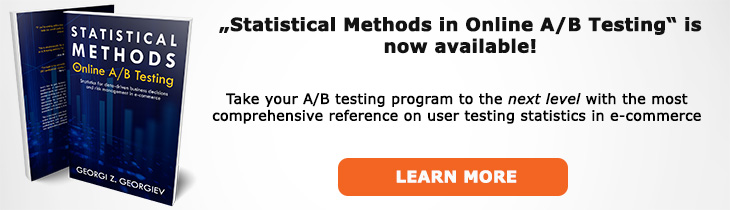# What does "Actual p-value" mean?

Definition of Actual p-value in the context of A/B testing (online controlled experiments).

## What is an Actual p-value?

Aliases: actual significance

An "actual p-value" is the actual probability of observing a test statistic as extreme or more extreme than the one observed during an A/B test. In a well-executed online controlled experiment (the statistical model is adequate) it coincides with the nominal p-value calculated and reported after the test, however in cases where there are violations of the assumptions of the significance test the actual p-value can be drastically different that the nominal one.

While in some cases it is possible to compute the actual p-value by respecifiying the statistical model in others we can only say that it is different that the nominal one and in which direction but it is not possible to calculate it precisely due to unknowns. An example of the former is when one has calculated a p-value for absolute difference but makes a claim about percent change. The model can be respecified with minimal hassle, other than loss of power. An example of the latter is cases of unaccounted peeking with intent to stop, especially when the exact number and timings of the peeks are unknown. In such a case a model can at best be approximately adequate or provide just a worst-case sceanrio.

## Related A/B Testing terms

Type I Error

Nominal p-value

Like this glossary entry? For an in-depth and comprehensive reading on A/B testing stats, check out the book "Statistical Methods in Online A/B Testing" by the author of this glossary, Georgi Georgiev.## Glossary Index by Letter

Select a letter to see all A/B testing terms starting with that letter or visit the Glossary homepage to see all.